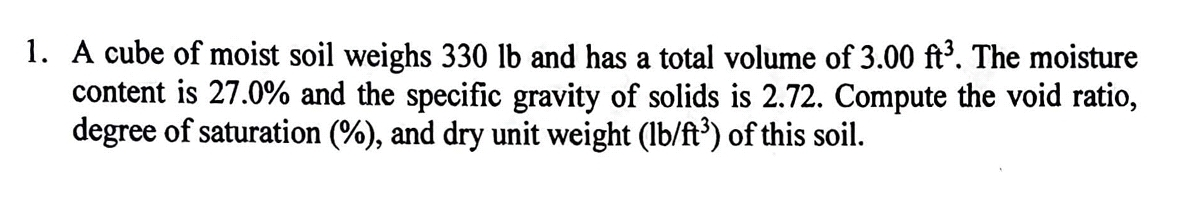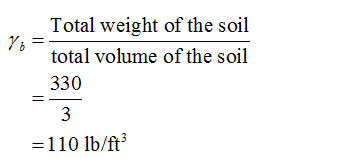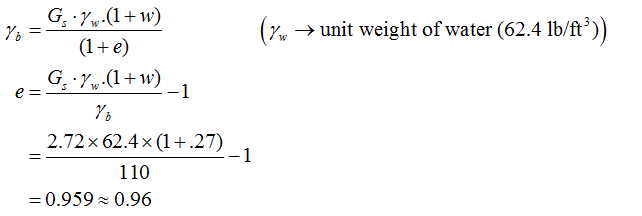# 1. A cube of moist soil weighs 330 lb and has a total volume of 3.00 ft³. The moisturecontent is 27.0% and the specific gravity of solids is 2.72. Compute the void ratio,degree of saturation (%), and dry unit weight (lb/ft³) of this soil.

Question
21 viewshelp_outlineImage Transcriptionclose1. A cube of moist soil weighs 330 lb and has a total volume of 3.00 ft³. The moisture content is 27.0% and the specific gravity of solids is 2.72. Compute the void ratio, degree of saturation (%), and dry unit weight (lb/ft³) of this soil. fullscreen
check_circle

Step 1

Given data:

Moist weight of soil (Wwet) = 330 lb

Total volume of Soil (V) = 3.0 ft3

Moisture content of soil or water content of the soil (w) = 27%

Specific gravity of the soil (Gs) = 2.72

Step 2

Calculate bulk unit weight of the soil:Step 3

Calculate the void ratio of the soil:...

### Want to see the full answer?

See Solution

#### Want to see this answer and more?

Solutions are written by subject experts who are available 24/7. Questions are typically answered within 1 hour.*

See Solution
*Response times may vary by subject and question.
Tagged in